﻿ Numerical Study of the Thermo-mechanical Behavior of 304L Stainless Steel Pipeline JunctionsPublications are Open
Access in this journal
Article Versions
Export Article
• Normal Style
• MLA Style
• APA Style
• Chicago Style
Research Article
Open Access Peer-reviewed

### Numerical Study of the Thermo-mechanical Behavior of 304L Stainless Steel Pipeline Junctions

Hicham Laribou , Abdelhalim Elbasset
International Journal of Physics. 2021, 9(3), 155-168. DOI: 10.12691/ijp-9-3-3
Received April 02, 2021; Revised May 03, 2021; Accepted May 11, 2021

### Abstract

The present paper is focused on the behavior of different junctions under the effect of internal pressure and temperature variation. The main junctions studied are of the T-junction and Y-junction type. The goal being to calculate the stress fields coupled to the temperature variation. This coupling realistically defines the thermo-mechanical behavior of the junction and in particular at the intersection where high stress concentrations are observed. The junction is an element widely used in industrial installations such as the cooling circuits of nuclear power plants which are subject to temperature fluctuations due to the mixture of hot fluids and cold these are called mixing zones. These fluctuations can lead to thermal or mechanical fatigue damage and cracking in the circuits causing leaks. Numerical modelling is carried out on the ANSYS calculation code based on the finite element method.

### 1. Introduction

Thermomechanical coupling in material failure has attracted great experimental and theoretical interest in recent years. Most of these studies are interested in the variation of the rate of elastic strain, the dynamic failure, and the thermal fatigue of cooling circuits, where the extreme loading rates can be the basis of the thermal softening of the material due to fairly high temperatures. In such problems, taking into account thermomechanical coupling is essential to understand the failure mechanisms.

The cooling circuits of nuclear power plants are subject to temperature fluctuations due to the mixing of hot and cold fluids in what are called mixing zones. These fluctuations can cause thermal fatigue damage in the circuits and in many cases cause leaks. Several incidents in the past have caused leaks and the shutdown of several power plants, both in France and abroad. In regards to nuclear power plants in the PWR (Pressurized Water Reactors) sector, several incidents have been attributed to thermal fatigue. In chronological order, they took place: in Germany in 1986, in Farley (USA) in 1987, in Tihange (Belgium) and Genkaï (Japan) in 1988, in Dampierre (France) in 1992. Finally, the most recent incident attributed to thermal fatigue took place in Civaux (France) in May 1998, when a water leak appeared on the Shutdown Reactor Cooling circuit (RRA) of the EDF (Electricité de France) plant. The crossing crack located at the circumferential weld of a mixing tee in Figure 1, is attributed to high cycles thermal fatigue. The surveys carried out on the damaged section did not reveal any significant manufacturing defects. A first functional analysis showed that the damaged piping area corresponds to a mixing area between two water flows whose temperature difference can reach 150°C depending on operating conditions. The flow conditions of this turbulent mixture result in fast temperature fluctuations at the pipe wall which lead to the beginning of fatigue damage characterized by isolated cracks located at the places of presence of singularities (welds) as well as by networks of cracks (crazing) in common areas 1.

An initial analysis of the Civaux incident and additional expertises concluded in thermal fatigue damage due to the turbulent mixture of hot and cold liquids. Taking this phenomenon into account at industrial level, not provided for by the design codes of PWR reactors, has resulted in the systematic replacement of all the mixing tees of the EDF fleet RRA circuits, as well as the definition of a simple method of identifying other areas of sensitive potential based on immediately available knowledge 2. The state of the art carried out during the definition of the project, as well as the first preliminary studies showed that specific investigations to this problem were necessary, both numerically and experimentally. In the field of thermo-hydraulics, the flow is complex and very dependent on the configuration of the mixing tee studied, as well as on the operating conditions (ratio between incoming flow and velocity flow). Tests under reduced thermal loads were carried out on models representative of the most stressed tees or connections. Measurements temperature in the fluid near the surface have made it possible to characterize the different stress profiles as a function of the configuration and operating conditions. At the same time, the experimental measurements obtained could be compared with the results of the corresponding numerical simulations.

• Figure 1. The tee of the cracked RRA mixture from the Civaux nuclear reactor

In terms of numerical simulation, the studies carried out on the mixing zones provided the opportunity to apply a L.E.S type modelling to an industrial component study (Large Eddy Simulation or Large Scale Simulation), the only one capable of producing a temporal signal of the local change in temperature, unlike the more traditional average field methods. The L.E.S. were coupled with the calculations of the evolution of thermal fields in the thickness of the piping, which constitute the input data for a thermomechanical calculation, by simulating the fluid / solid heat transfer 3. Also, the NRG group (Nuclear Research and Consultany Group) have experimentally and theoretically studied thermal fatigue in the mixing zones (T-junction) of a nuclear reactor. The simulation test is based on an experimental device, which was used before validating the CFD (Computational Fluid Dynamics) model for adiabatic flow as shown in Figure 2 with two perpendicularly connected pipes.

• Figure 2. T-junction experimental device

Structural temperatures resulting from CFD analysis were used as thermal charges in finite element analysis 4. We also find the work of 5, where the authors of this research present the finite element analysis of the constraints on T-junction with the I-DEAS program. The main objectives of this study are to predict the distribution of stresses and strains of T-junctions and to study the flow of fluids effect and gas-liquid on the stress. They showed that the highest value of stress occurred at the intersection zone of the junction. The Von Mises stress at this area was 221.95MPa, this value exceeded the yield strength of the material and the displacements are higher in this area 0.68mm. In the field of material behaviour, the main gaps identified at the start of the project were related to the limits of knowledge on the fatigue behaviour of stainless steels in the field of large numbers of cycles (beyond 105 cycles), as well as on the possible specificity of thermal fatigue. 6 concluded, based on thermomechanical fatigue tests on V.E.R. that the thermal fatigue of AISI 304L steel could be likened to mechanical fatigue for a temperature below 300°C and a service life of up to 105 cycles. A vast experimental campaign on test specimens made it possible to specify the fatigue strength of 304L steel in the range between 105 and 107 cycles as a function of numerous parameters such as the temperature, the level of average stress, the control mode, the surface state, the pre-hardened state or not of the material, the medium (water or air) 7.

• Figure 3. Endurance test on a steel mock-up (mixing tee)

The main goal of this study is to determine by numerical modelling the thermomechanical behaviour of two types of junctions (T-junction e Y-junction). These junctions are used in cooling circuits, condensate under loading conditions mechanical and thermal. The study will mainly concern the calculation of stresses and strains in these junctions and particularly at intersections under the effect of varying pressures and temperatures. The code ANSYS is used in this study. The finite element method is applied to discretize the constituent equations as implemented in ANSYS.

### 2. Fundamental Theory and Method

With the development of high performance computer and advanced numerical techniques, FE analysis is widely accepted and considered as a practical and effective research tool to study the behavior of different junctions under the internal pressure effect and the variation of the temperature. The fundamental theory and FE analysis method used in this study are presented as follows, although the study will be focused on the case of a homogeneous and isotropic elastic material.

2.1. Temperature Distribution Equations

In this problem, we will suppose that the fluid temperature field is uniform on the internal surface of the solid (junction or pipe). We make the assumption of a purely radial diffusion of heat through the wall of the piping, which can be thermally assimilated to a plate plane. The fluid-structure coupling is taken into account by means of an exchange coefficient H independent of the frequency. Under these assumptions the resolution of the thermal problem, i.e. determining the temperature fields in the internal piping surfce amounts to solving the one-dimensional heat equation for a homogeneous and isotropic material:(1)

Where T is temperature and ρ is the solid density, λ is thermal conductivity, CP is the specific heat capacity at constant pressure and q is the power density released, and.

2.1.1. Differential Form

As known, Solving a thermal problem consists in looking for a temperature field T(x,y,z;t) at any point of the solid such that: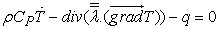(1a)

With the following boundary conditions:(2)

We introduce now the quantity r(T) defined by:(3)

r(T) is called the residue of equation (1.a), r(T) is zero if T is a solution of equation (1.a) and different from 0 otherwise.

• Figure 4. Boundary conditions in the case of T and Y junction

2.1.2.Weak Integral Form

To solve equation (1.a) by the finite element method, we use the weighted residual method in Galerkin's formula 12. Multiply equation (1.a) by an arbitrary function T and integrate over the domain V:(4)

The T function is called a weighting function (or function test.

Using Ostrogradski's theorem, the boundary conditions equations (2) and, we obtain the weak integral formulation of a thermal problem:(5)

The functions T and T must be sufficiently regular for the above expressions to have a meaning.

The function T is called the virtual temperature field, h is the the convection coefficient and Tf is the fluid temperature. In equation (4) the function T must be differentiable twice and once in equation (5). These equations are called the strong integral form and weak integral form of the differential equation (1.a), respectively. Under certain regularity conditions, formulations (1.a) and (5) are equivalent.

2.1.3. Temperature Field in Discretized Form

The analytical solution of equation (1) is generally inaccessible. One is thus led to seek an approximate solution by a numerical method: the finite element method. This method is a special case of the Galerkin method: the temperature field and the test functions belong to the same finite dimensional space 13. We consider a domain V decomposed into subdomains Ve of simple geometrical form (the elements) connected between them at points called nodes. The temperature field T (x, y, z; t) in element (e) has the expression 14: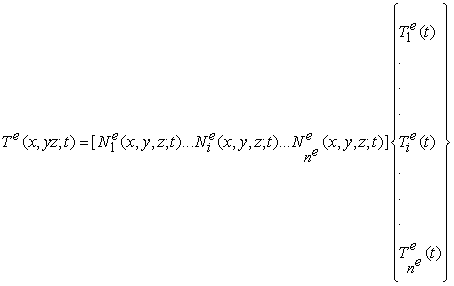(6)

Where: ne is the number of nodes of the element, the functions 𝑁𝑖𝑒(𝑥,𝑦,𝑧;t) are the elementary interpolation functions, the matrix [Ne(x,y,z)]is the elementary interpolation matrix and the vector {Te(t)} gathers the temperatures of the nodes of the element (e).

Therefore the temperature field T (x,y,z;t) is expressed over the whole domain V as: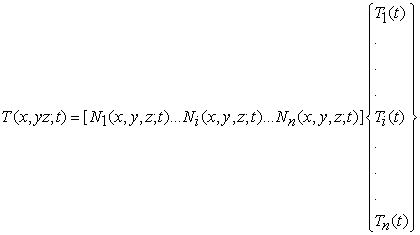(7)

Where: n is the number of nodes of the mesh, the functions (𝑥,,𝑧;t) are the interpolation functions (or shape function), the matrix [N(x,y,z)]is the interpolation matrix and the vector {T(t)} is the vector of nodal temperatures.

Note also that the interpolation functions verifying the relations: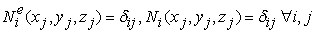Where (xj,yj,zj) are the coordinates of j node and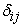is the Kronecker coefficient.

2.1.4. Partition of Freedom Degrees

We partition the freedom degrees into unknown {TL} and {TP} known temperatures.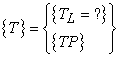(8)

Where the vector {TP} gathers the temperatures (known) of the nodes located on the surface S. This partition induces a partition of the interpolation matrix 15: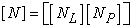(9)

Hence the expression of T and T:(10)

2.1.5. Discretization of the Weak Integral Form

From the expression of the temperature field T on the domain V: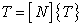(11)

We deduce that:(12)

And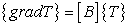(13)

With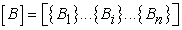(14)

For a spatial problem, {Bi} is written in the orthonormal frame {x, y, z}:(15)

Similarly, we have: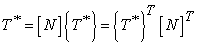(16)

Hence:(17)

By replacing relations in equation (5), it comes: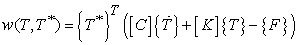(18)

Where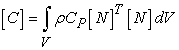(19)(20)(21)

[C] is the thermal capacity matrix (J / K).

[K] is the thermal conductivity matrix (W / K).

{F} is the vector of the nodal flows (W).

{T} is the vector of the nodal temperatures (K).

By construction, the matrices [C] and [K] are symmetrical, and the partition of the degrees of freedom induces a partition of [C], [K] and {F}: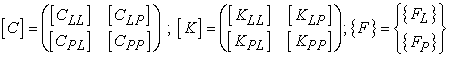(22)

The discrete form of a thermal problem is finally written to find {TL (t)} such that: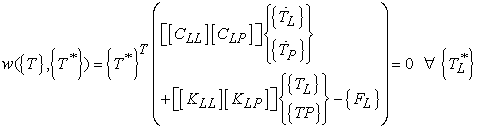(23)

With the initial condition {TL (t0)} = {TL, 0}.

The unknown nodal temperatures {TL(t)} are therefore the solutions of the equation:(24)
2.2. Thermal Strain Distribution and Isotropic Thermoelasticity

We adopt the hypothesis of homogeneous and isotropic linear elasticity, an infinitely long chopped tube (plate approximation, i.e. thickness << internal diameter) uniformly stressed in the internal skin by a fluid temperature field, with its free ends to expand in the axial direction. The reference temperature (absence of thermal strains, zero stress state) is moreover taken equal to the temperature at time t0. Strain can also be caused by a change in temperature 16. In the isotropic case and in the absence of constraint, we have:(25)

Wherethe coefficient of linear thermal expansion and ∆T is corresponds to temperature change (T-T0). In most crystal structures, a change in temperature does not induce shear. When both a stress and a change in temperature are imposed, the total strain {𝜀𝑡𝑜𝑡} ={𝜀𝑒𝑙}+{𝜀th} is the sum of an elastic strain 𝜀𝑒𝑙 and a thermal strain 𝜀th (to which a plastic strain can still be added if the limit elasticity is exceeded, as well as piezoelectric deformations in the presence of an electric field or ferromagnetic in the presence of a magnetic field). The complete Hooke's law (valid only in the case of thermoelastic strain) is then written: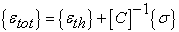(26)

Where {𝜎} is he stress vector, [C] is the stiffness matrix which is written for an Isotropic material as: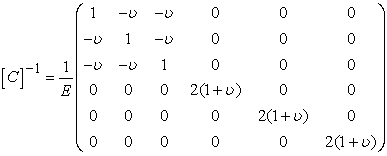(27)

Where E is the Young's modulus and υ is Poisson's ratio. This leads us to: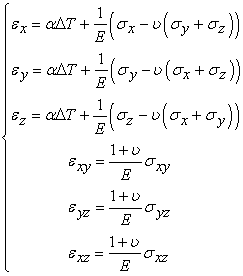(28)
2.3. Thermal Stress Distribution and Isotropic Thermoelasticity

From the expression of the temperature field in the piping determined in the previous paragraph and from the linear elasticity relation (Hook’s law) in continuous solid 17, it is possible to determine analytically the components of the stress tensor as: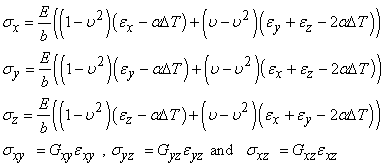(29)

Where,.

Thermal stresses 18, sometimes wrongly called "thermal stresses", come from the fact that a material subjected to a change in temperature is constrained in such a way that it cannot deform freely. In this case, the thermal deformation is compensated by an elastic deformation. It is thus indeed the elastic deformation (which corresponds to a displacement of the atoms compared to their position of equilibrium, which has changed with the temperature) which is at the origin of the stress, it is only indirectly that the change in temperature induces a constraint. A free object deforms under the effect of a change in temperature, without any stress appearing 19.

### 3. Numerical Modelling Procedure

3.1. Material and Working Conditions

The Material used in this study is austenitic 304L stainless steel, the physical characteristics of which are given in Table 1 at different temperatures.

Table 2 shows the characterization tests in monotonic traction which were carried out at temperatures: ambient, 150°C and 300°C on cylindrical specimens with a diameter of 10mm taken in the longitudinal direction (L).

In this study, the model shown, in both Figure 5 and Figure 6 is a cylindrical main pipe (tank) is drilled radially by a small pipe at a point on its axis at a distance from the use of the large pipe. The interior of the reservoir contains a fluid at a temperature (232°C) at a pressure of 2.2bar. The fluid in the small pipe is subjected to a pressure of 2.5bar and a temperature of 38°C. The convection coefficients in the tank and the pipe are respectively 1420Watts/m².°C and 2380Watts/m².°C. The value of the heat transfer coefficient by convection depends on the nature of the fluid, its temperature, its speed and the geometric characteristics of the solid/fluid contact surface. In Figure 5 and Figure 6 are presented the junction model used in our computation. All dimensions are given in meters.

• Figure 5. T-junction dimensions
• Figure 6. Y-junction dimensions
3.2. The Initials Conditions and the Boundary Conditions

The T and Y junctions were retained on three ends by six degrees of freedom (3 translations and 3 rotations) and therefore cannot move independently in any direction. The initial conditions and the boundary conditions used, are as follows: (u, v and w the components of displacements in the three directions x, y and z respectively).

• Figure 7. Initials conditions and limits of Y and T junction
3.3. Mesh Quality and Chosen Path

The quality of the mesh plays a significant role in the precision and the stability of numerical computation. Thus, attributes such as the distribution of knots, the smooth nature and the obliquity of the elements are very important. For this study, the triangular element was suitable due to the excellent results compared to the rectangular element. However, triangular elements have the limitation of the mesh in the T-junction area 20 and 21.

• Figure 8. T-junction Mesh contains 8939 element and 11046 nodes
• Figure 9. Y-junction Mesh contains 8650 element and 11687 nodes

To specify the trajectory, we select three nodes on the internal surface of the two junctions (Figure 10). On this path we calculate the elastic strain, the thermal strain and the thermoelastic coupling.Figure 10. Chosen path for: a) T-junction, b) Y-junction

### 4. Results and Discussion

4.1.1. T-Junction

The nodal distribution of temperature and thermal strain are shown in Figure 11 and Figure 12. It is observed that the temperature variation begins as it approaches the intersection zone, where the hot and cold fluids meet.

• Figure 11. Nodal distribution of temperature
• Figure 12. Thermal strain distribution

The use of all the results obtained made it possible to draw the two graphs shown in Figure 13 and Figure 14.

• Figure 13. Variation of temperature following the T-junction path

The Figure 13 and Figure 14 show the evolution of the temperature and the thermal strain in the three directions (x, y and z) as a function of the distance of the path defined in Figure 10. Thus, we see that the distribution of the temperature is homogeneous in all three directions. The temperature starts with a stable value T=232°C and approaches the intersection where it gradually decreases until T=121.81°C (node N°36) where the thermal strain has been determined Ɛth=0.18461x10-2[%] which has an important significance because it is at this distance (0.21m) that the maximum elastic strain was also calculated. The temperature stabilizes at the value of T=38°C.

• Figure 14. Variation of thermal strain following the T-junction path

4.1.2. Y-Junction

The temperature distribution and thermal deformation are shown in Figure 15 and Figure 16, we also observe that the temperature variation begins when approaching the intersection zone.

In the same way, the thermal strain recorded at the temperature T=115.75°C (node N°2866) having for value Ɛth=0.17637x10−2[%] at the same distance (0.21m). The Figure 17 and Figure 18 show the temperature and thermal strain evolution.

• Figure 15. Nodal distribution of temperature
• Figure 16. Thermal strain distribution
• Figure 17. Variation of temperature following the T-junction path
• Figure 18. Variation of thermal strain following the Y-junction path

4.1.3. Comparison between T and Y Junction

To see the influence of the types of the junctions on the temperature distribution along the three axes, we considered the curves presented in Figure 19 and 20. We notice a slight difference between the T and Y junction at the same distance of 0.21m. What is visible in Table 3. Figure 21 shows the evolution of thermal strain as a function of the two junctions temperature.

• Figure 19. Temperature distribution
• Figure 20. Thermal strain distribution
• Figure 21. Evolution of thermal strain as a function of temperature
4.2.1. T-Junction

In this case, the calculations made it possible to note that the greatest strain is located at the node N°36 according to x direction. Figure 22 shows the evolution of the stress and the strain of the same junction. We can notice also, that the maximum of the stress is reached according to the direction x. What is clearly visible in the Figure of the elastic strain Figure 23.

• Figure 22. Evolution of stress in x, y and z directions for T-junction
• Figure 23. Evolution of elastic strain in x, y and z directions for T-junction

4.2.2. Y-Junction

In the same way, for the Y junction, the greatest deformation is located at node N°2866 (along x). Figure 24 gives the evolution of stresses. We can also notice that the maximum of the stress is reached according to the direction x. What is clearly visible in the Figure of the elastic deformation Figure 25.

• Figure 24. Evolution of stress in x, y and z directions for Y-junction
• Figure 25. Evolution of elastic strain in x, y and z directions for Y-junction

4.2.3. Comparison between T and Y Junction

Table 4 shows the maximum results of the stresses and strains along the three axes of the two junctions (T and Y). It is recorded that the maximum in stress, strain is obtained in the Y-junction and along the x direction which is shown in Figure 26 and Figure 27.

• Figure 26. Evolution of elastic strain in x, y and z directions for Y-junction
• Figure 27. Evolution of elastic strain in x, y and z directions for Y-junction
4.3.1. T-Junction
• Figure 28. The total strain of T-junction along the three axes

According to the results obtained, we have that the greatest strain is located at the node N ° 36 following x directions. Figure 28, shows the evolution of the total deformation of the same junction. One can notice that the maximum of the strain is following the same direction.

4.3.2. Y-Junction

In the same way, for the Y junction, the greatest deformation is located at node N°2866 (along x). Figure 29 gives the evolution of the total deformation of the same junction. We can also notice that the maximum of the strain is reached according to the x direction.

• Figure 29. The total strain of Y-junction along the three axes

4.3.3. Comparison between T and Y Junction

Unlike thermal deformation, the Y junction deforms much more than that of T. Table 5 shows the maximum results of the total deformations along the three axes of the two junctions (T and Y). Figure 30 represents the comparison of the total deformations between the two junctions (T and Y) in the direction (x).

• Figure 30. Comparisons of total strain between T and Y-junction in the x direction
4.4. Elastic, Thermal and Thermo-elastic Strain

Table 6 groups together the different values of the elastic, thermal and thermoelastic strains. Note that the Y junction deforms more than that of T. The contribution of thermal strain is relatively high in the total strain. The comparison of these values is made at the same distance (0.21m). Figure 31 and Figure 32 show the comparison between the deformations of the T and Y junctions according to the chosen path (presented in Figure 10).

• Figure 31. Elastic, thermoelastic and total strain for T-junction in the x direction
• Figure 32. Elastic, thermoelastic and total strain for Y-junction in the x direction
4.5. The VON MISES Stress

The computation of the Von Mises stresses was carried out for the two junctions following the path presented in Figure 10. For the T junction it is constant that the maximum of the stress is reached at node 52 having a value of 1.5694x108[Pa]. On the other hand, at junction Y, the maximum is recorded at node 2882 with a value of 1.29490x108[Pa]. It is observed that the junction T is the most stressed. Table 7 shows the maximum results of the principal and equivalent stresses for the two junctions (T and Y) loaded at the same internal pressure, which leads to calculate principal stresses only (.𝜎1, 𝜎2 𝑒𝑡 𝜎3). For the T-junction, we note symmetry in the distribution of the stresses with respect to the axis of symmetry. On the other hand, this symmetry disappeared in the Y-junction. These values do not exceed the elastic limit of the material.

• Figure 33. Principal and equivalent stresses following the T-junction path
• Figure 34. Principal & equivalent stresses following the Y-junction path
• Figure 35. Comparison of the Von Mises stress between T and Y junction

Figure 35 gives a comparison of the two junctions as a function of the Von Mises stress. We see that the shape of the two curves is almost identical except that the T junction is the most heavily loaded.

### 5. Conclusion

In conclusion of this study, we can affirm that the thermomechanical modeling of the various junctions is above all a safety character. Indeed, the behavior of the junction under loading conditions (pressure and temperature) characterizes the reliability and safety of industrial installations. The choice of a particular type of junction is subject to the calculations of the various strains. In our study, two types of junction were calculated, the stress concentration is located at the intersection. The T-junction behaves favourably with respect to the Y-junction. The elastic strain in the T junction is 0.42% on the other hand that of Y-junction is 0.62%. The thermal strain in the T junction is 0.18% and 0.17% in the Y-junction. The total strain (thermo-elastic) in the T-junction 0.61% on the other hand that of Y-junction is 0.79%. The Von Mises stress for the T-junction is 156.94MPa. On the other hand, at the Y-junction is 129.49MPa, these values do not exceed the elastic limit of the material. The results presented in this study relate to the modelling of seamless junctions. Looking ahead, we recommend: consider the behaviour of weld beads, test other types of junctions and use a new materials (composite materials).

### References

  C. Faidy. La fatigue thermique dans les centrales nucléaires. Enseignement du retour d’expérience. In Proc. of International Symposium on Contribution of Materials Investigations to the resolution of problems encountered in pressurized water reactors, pp 847-857, 2002. In article  O. VOLTE, F. BEAUD, “Method for identification of fluid mixing zones subject to thermal fatigue damage. ”, int. conf. on residual fatigue life and life extension of in-service structures, May 30 – June 01, (2006), Paris. In article  T. PASUTTO, C. PENIGUEL, M. SAKIZ, J.M. STEPHAN, “Chained computations using an unsteady 3D approach for the determination of thermal fatigue in a T-junction of a PWR nuclear plant” ASME – PVP Conference, July 2005, Denver. In article  M.H.C. HANNINK, A.K. KUCZAJ, F.J. BLOM, J.M. CHURCH AND E.M.J. KOMEN, “A coupled CFD-FEM strategy to predict thermal fatigue in mixing tees of nuclear reactors” Nuclear Research and Consultancy Group (NRG) ,(2006), The Netherlands. In article  CHAKRAPANI BASAVARAJU, “Thermal Stresses at Dissimilar Pipe-Stanchion Interfaces” ASME Pressure Vessels and Piping Conference, (2004), Bechtel Corporation ,Frederick, Maryland. In article View Article  N. HADDARD, “Fatigue thermique d’un acier inoxydable austénitique 304L: simulation de l’amorçage et de la croissance des fissures courtes en fatigue isotherme et anisotherme”, Thèse ENSMP, (2003). In article  J.C. LEROUX, F. CURTIT, J.M. STEPHAN, “Study of thermal and mechanical fatigue of 304L stainless steel: comparison between tests on mockups and test on standard specimen”, Int. Fatigue cong. Fatigue 2006, May 14-19, Atlanta. In article  HAYASHI M., ENOMOTO K., SAITO T., MIYAGAWA “Development of thermal testing apparatus with BWR water environment and thermal fatigue strength of stainless steel, in : Fracture mechanics applications”, 1994, ASME. In article  V. DOQUET, S. TAHERI, “Effet d'un pré-écrouissage ou d'un sur-écrouissage sur la durée de vie en fatigue de divers aciers à contrainte ou déformation imposes”, Revue Française de Mécanique, (2000), pp. 35-40. In article  V. MAILLOT, “Amorçage et propagation de réseaux de fissures de fatigue thermique dans un acier inoxydable austénitique de type X2 CrNi18-09 (AISI 304L)”, Thèse ENSMP, (2004). In article  F. CURTIT, J.M. STEPHAN “INTHERPOL Thermal fatigue tests”, ASME – PVP Conference, July 2005, Denver. In article View Article  A. FISSOLO and al “Advances in thermal fatigue investigations performed in CEA for French PWR pipings - Third International Conference on fatigue of Reactors Components” October 3-6 (2004) – Seville, Spain. In article  Le Dret H. (2013) La méthode de Galerkin. In: Équations aux dérivées partielles elliptiques non linéaires. Mathématiques et Applications, vol 72. Springer. In article View Article  S. CHAPULIOT , C. GOURDIN , T. PAYEN , J.P. MAGNAUD , A. MONAVON “Hydro-thermal-mechanical analysis of thermal fatigue in a mixing tee” Universities Pierre et Marie Curie, 2 April 2004-France. In article View Article  TOSHIHARU MURAMATSU “Evaluation Of Thermal Striping Phenomena At a Tee Junction Of LMFR Piping Systems With Numerical Methods, Thermohydraulic Caculations”, Japan Nuclear Cycle Development Institute, 1999, Japan. In article  RICHARD NEKVASIL, “Influence Of Temperature Differences Of Mixed Streams Upon T-Junction Damage” University of Technology, February 21, 2008-Brno. In article  M.D. Xue , D.F. Li , K.C. Hwang “Theoretical stress analysis of intersecting cylindrical shells subjected to external loads transmitted through branch pipes” department of engineering mechanics, tsinghua university, 2 July 2004- China. In article  BENHAMADOUCHE S., SAKI M., PENIGUEL C., STEPHAN J.M. “Presentation of a New Methodology of Chained Computations using Instationary 3D Approaches for the Determination of Thermal Fatigue in a T-Junction of a PWR Nuclear Plant”, In 17th International Conference structural Mechanics in Reactor Technology (SMIRT 17), Prague 2003. In article  H.-Y.LEE, J.-B.KIM, B.YOO. “Tee-Junction Of LMFR Secondary Circuit Involving Thermal, Thermomechanical And Fracture Mechanics Assessment On A Striping Phenomenon” Korea Atomic Energy Research Institute, 1999, Republic Of Korea. In article  ANSYS Finite Element Analysis Program "thermal and structural Analysis Guide” Version 11. In article  SOLIDWORKS code de design industrial, Version 7. In articleThis work is licensed under a Creative Commons Attribution 4.0 International License. To view a copy of this license, visit http://creativecommons.org/licenses/by/4.0/